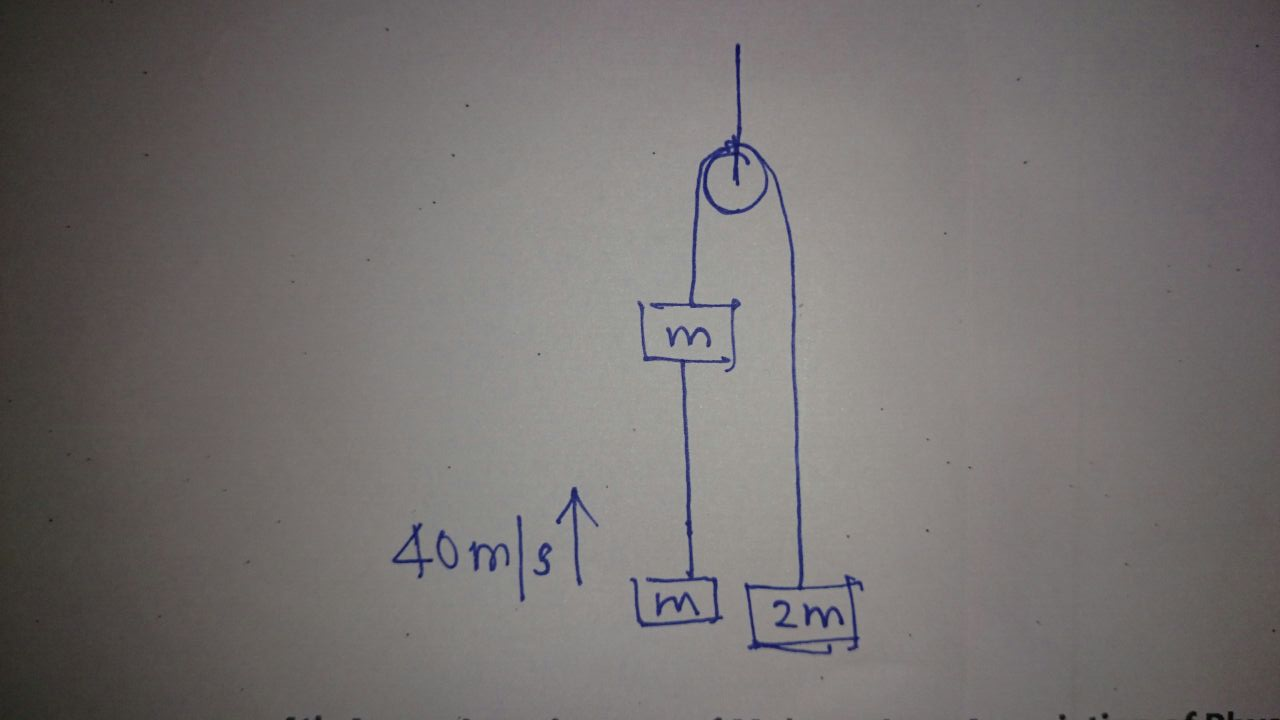# Pulley Challenge!A mass of $2m$ kg is suspended from the string on one side while two masses of $m$ kg are on the other side as shown.

The system starts at rest. Suddenly, the lower mass of $m$ kg is flicked up with velocity 40 m/s.

After how many seconds will the rope be taut again?

Details

• $g = 10\text{ m/s}^2$
• Assume the rope is very long and the masses do not collide.
×